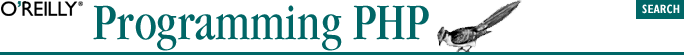home | O'Reilly's CD bookshelfs | FreeBSD | Linux | Cisco | Cisco Exam## 2.4. Expressions and Operators

#### Table 2-3. PHP operators

P

A

Operator

Operation

19

N

`new`

Create new object

18

R

`[`

Array subscript

17

R

`!`

Logical NOT

R

`~`

Bitwise NOT

R

`++`

Increment

R

`--`

Decrement

R

(int), (double), (string), (array), (object)

Cast

R

`@`

Inhibit errors

16

L

`*`

Multiplication

L

`/`

Division

L

`%`

Modulus

15

L

`+`

L

`-`

Subtraction

L

`.`

String concatenation

14

L

`<<`

Bitwise shift left

L

`>>`

Bitwise shift right

13

N

<, <=

Less than, less than or equal

N

>, >=

Greater than, greater than or equal

12

N

`==`

Value equality

N

!=, <>

Inequality

N

`===`

Type and value equality

N

`!==`

Type and value inequality

11

L

`&`

Bitwise AND

10

L

`^`

Bitwise XOR

9

L

`|`

Bitwise OR

8

L

`&&`

Logical AND

7

L

`||`

Logical OR

6

L

`?:`

Conditional operator

5

L

`=`

Assignment

L

+=, -=, *=, /=, .=, %=, &=, |=, ^=, ~=, <<=, >>=

Assignment with operation

4

L

`and`

Logical AND

3

L

`xor`

Logical XOR

2

L

`or`

Logical OR

1

L

`,`

List separator